RS Aggarwal Class 7 Solutions Chapter 3 - Decimals

RS Aggarwal Class 7 Chapter 3 - Decimals Solutions Free PDF

A number which contains a decimal point can be defined as a Decimal point and the place value is the system for helping in categorizing a number into the ones, hundreds or thousand. Decimal numbers are separated by the decimal number according to the decimal system. The number system for decimals increases in place value from right to left and the numbers after the decimal point decreases in value.

An example of a simple decimal is 6.321 where,

• 3 is in the hundreds position
• 2 is in the tens position
• 1 is in the ones position

Check out the RS Aggarwal Class 7 Solutions Chapter 3 Decimals available below: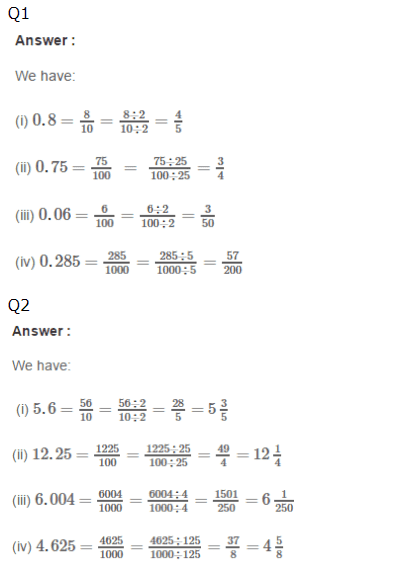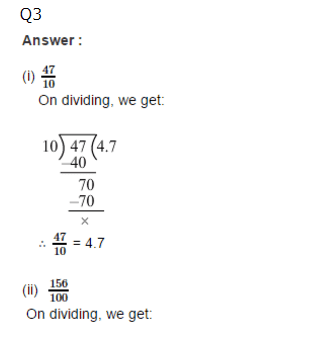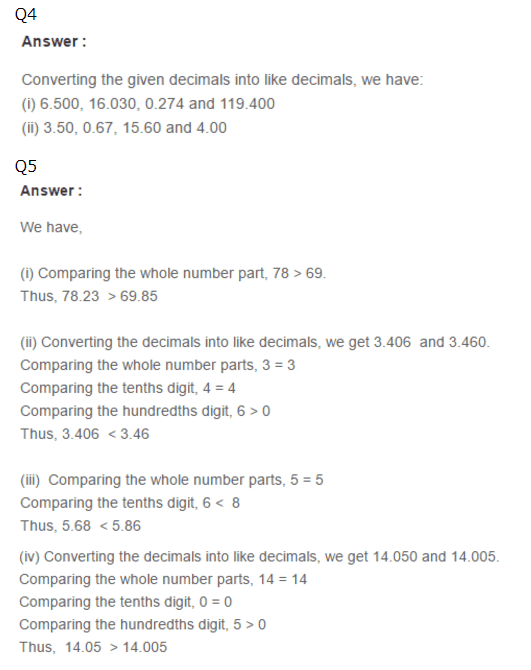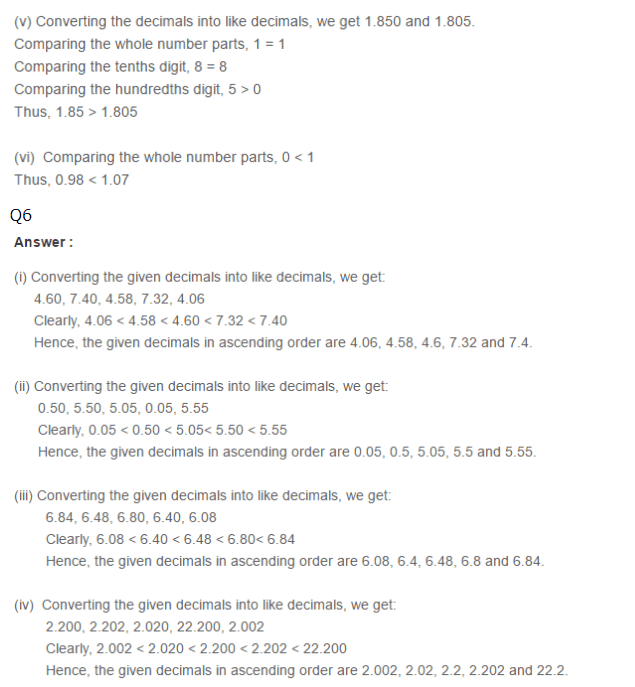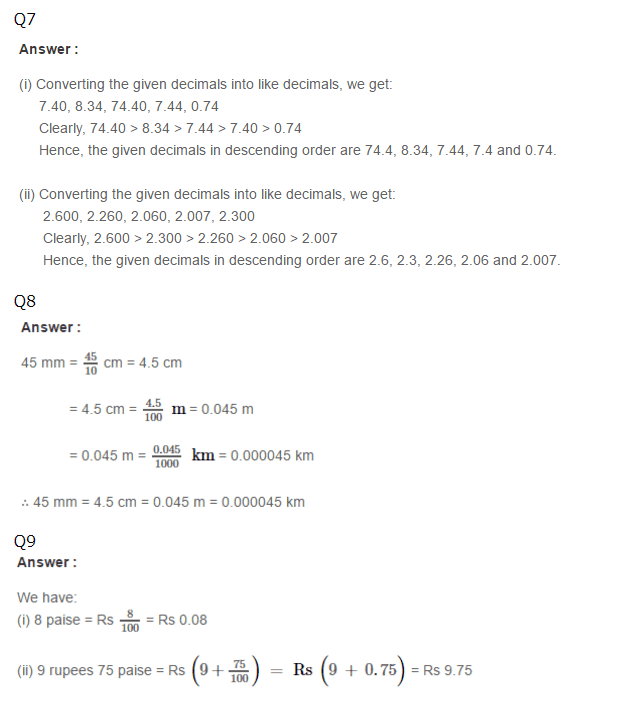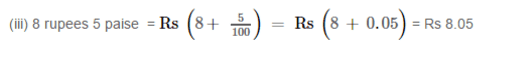Practise This Question

Which of these are components of a pistil?

I: Style

II: Anther

III: Stigma

IV: Ovary